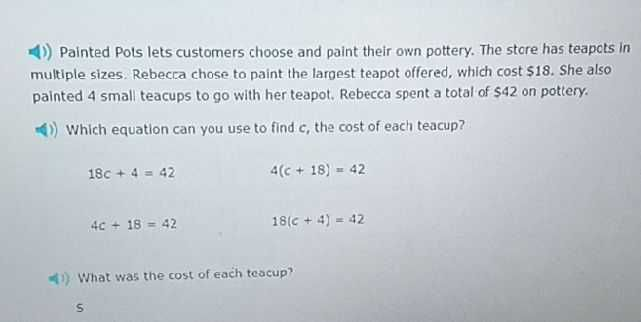### ¿Todavía tienes preguntas de matemáticas?

Pregunte a nuestros tutores expertos
Algebra
Pregunta(1)) Painted Pots lets customers choose and paint their own pottery. The store has teapcts in multiple sizes. Rebecca chose to paint the largest teapot offered, which cost $$\ 18$$ . She also painted $$4$$ small teacups to go with her teapot. Rebecca spent a total of $$\ 42$$ on pottery.

$$18 c + 4 = 42$$

$$4 c + 18 = 42$$

$$4 ( c + 18 ) = 42$$

$$18 ( c + 4 ) = 42$$

$$5$$